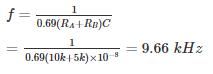Courses

# Analog Electronics - 8

## 10 Questions MCQ Test RRB JE for Electronics & Communication Engineering | Analog Electronics - 8

Description
This mock test of Analog Electronics - 8 for Electronics and Communication Engineering (ECE) helps you for every Electronics and Communication Engineering (ECE) entrance exam. This contains 10 Multiple Choice Questions for Electronics and Communication Engineering (ECE) Analog Electronics - 8 (mcq) to study with solutions a complete question bank. The solved questions answers in this Analog Electronics - 8 quiz give you a good mix of easy questions and tough questions. Electronics and Communication Engineering (ECE) students definitely take this Analog Electronics - 8 exercise for a better result in the exam. You can find other Analog Electronics - 8 extra questions, long questions & short questions for Electronics and Communication Engineering (ECE) on EduRev as well by searching above.
QUESTION: 1

###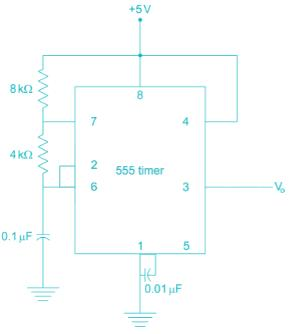Which is the frequency of the given 555 timer?

Solution: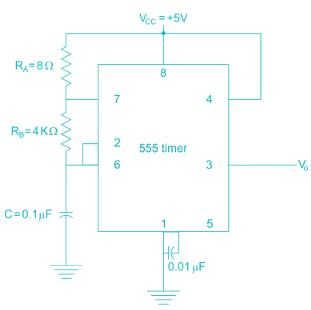The waveforms will be like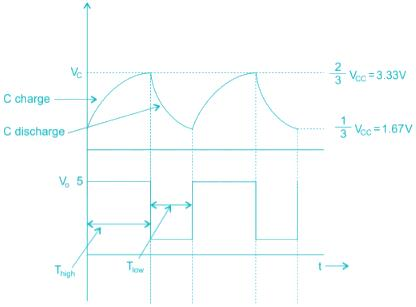The charge of the capacitor varies between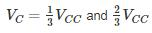When capacitor is getting charged, output V0 is high

When capacitor is getting discharged, output V0 is low

The time period of o/p, T = Thigh + Tlow

In Thigh, i.e. charging time, both RA& RB are in play.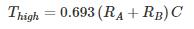In Tlow, i.e. discharging time,

Tlow, i.e. discharging time,

Tlow = 0.693 RBC

T = Thigh + Tlow

= 0. 693(RA + 2RB)C

= 0.693 (8 + (2 × 4) × 103 × 0.1 × 10-6)

= 0.693 × 16 × 103 × 10-7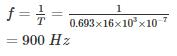*Answer can only contain numeric values
QUESTION: 2

### In the above monostable multivibrator circuit above given C = 10 nF. If the frequency of output pulse is 100 kHz the resistor value is _________ kΩ

Solution:

The pulse duration in mono-stable multivibrator using 555 timer is T = RC In3

10-5 = 1.1 × R × 10 × 10-9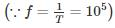R = 909.1 Ω

≃ 0.91 kΩ

QUESTION: 3

### For the 555 timer circuit, if RA = RB then

Solution:

The ON time of 555 timer is TON = RA + RB and OFF time TOFF = RB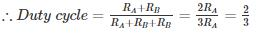≃ 66% > 50%

QUESTION: 4

A dc voltage of 380 V with a peak ripple voltage not exceeding 7 V is required to supply a 500 Ω load. Find out the inductance required.

Solution:

We know that the ripple factor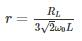Where r = RMS value of AC component/DC component

RMS value of AC component = 7/1.414 = 4.95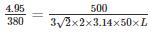L = 28.8 H

*Answer can only contain numeric values
QUESTION: 5

A shunt regular is shown in the figure has a regulated output voltage of 10 V. Given Vz = 9.3, VBE = 0 .7 V, β = 49. Neglecting the current through Resistor RB the ratio of maximum power dissipated in the transistor to the maximum power dissipated in the Zener diode is ________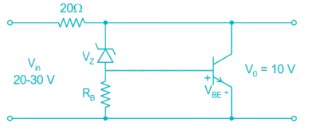Solution: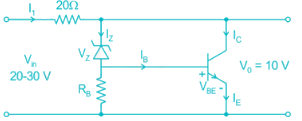Maximum input current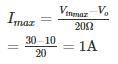The Base current is equal to current flowing through Zener diode

Vz = IB

→ Ic = βIB = βIZ

I1 = βIZ + Iz

1A = (49 + 1) Iz

Iz = 20 mA

∴ Power dissipated by the Zener diode Pz = VzIz

= 9.3 × 0.02 = 186 mW

Power dissipated by the Zener diode Pc = Ic × Vc

IC = 99Iz; VC = VO

⇒ PC = 0.98 × 10 = 9.8 W
∴ The ratio =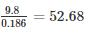QUESTION: 6

For the circuit shown, the value of C = 1 nF, the value of RA & R such that oscillation frequency of 100 kHz and duty cycle frequency of 75% is obtained at the output is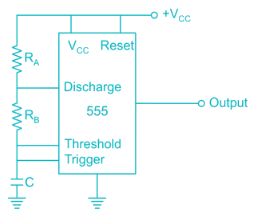Solution:

T = 0.69 C (RA + 2RB)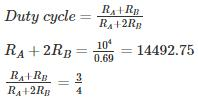4RB = 14452.75

RB = 3623

3.6 K

RA = 7.2 K

*Answer can only contain numeric values
QUESTION: 7

The frequency of oscillation of the astable multivibrator circuit given below is ______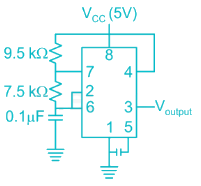Solution:

The time period of astable multivibrator given is

T = 0.693 (RA + 2RB) C

Where RA = 9.5 kΩ RB = 7.5 kΩ

C = 0.1 μF

Frequency of oscillation is given by F = 1/T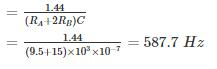QUESTION: 8

For the given circuit, what is the value of power dissipated in zener diode ?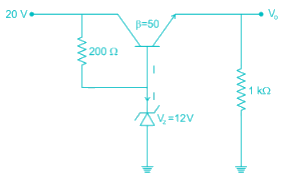Solution:

The given circuit is of a voltage regulator, hence the output voltage will remain fixed due to zener diode.
V0 = VZ – VBE = 12 − 0.7 = 11.3V
VCE = 20 – 11.3 = 8.7V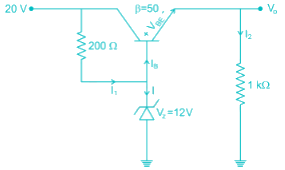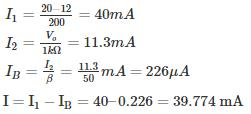Hence power dissipated in zener diode is Pdis = V× I = 477.3 mW

*Answer can only contain numeric values
QUESTION: 9

The output voltage of the voltage regulator is constant at 18.5 V. Assuming the op-amp and Zener diode are ideal, the maximum power dissipated in the transistor is ______ mw. The transistor β is very high.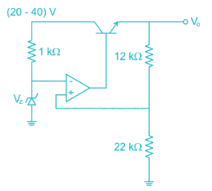Solution: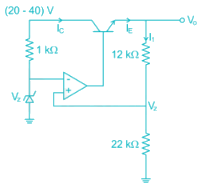Due to virtual short, the voltage at inverting terminal is Vz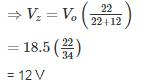Then the current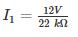≃ 0.545 mA

Since op-amp is ideal no current is drawn by it,

⇒ IC ≃ IE = I1

VCE = (20 ~ 40) V – 18.5 V

VCEmax = 21.5 V

Power dissipated (maximum) = 21.5 × 0.545 mA

≃ 11.72 mW

*Answer can only contain numeric values
QUESTION: 10

For the timer circuit shown below the frequency of oscillation is __________ kHz.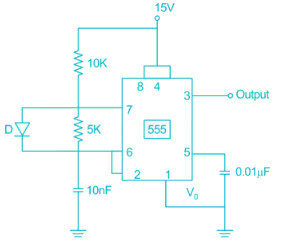Solution:

In the given circuit R= 10 kΩ RB = 5 kΩ C = 1 nF

The frequency of oscillation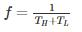Normally the duration of 'high' of pulse is TH = C(RA + RB)ln 2, but the diode bypasses the lower resistance RB. And the capacitor is charged only through RA.

Hence TH is = 0.69RAC and TL = 0.69RBC